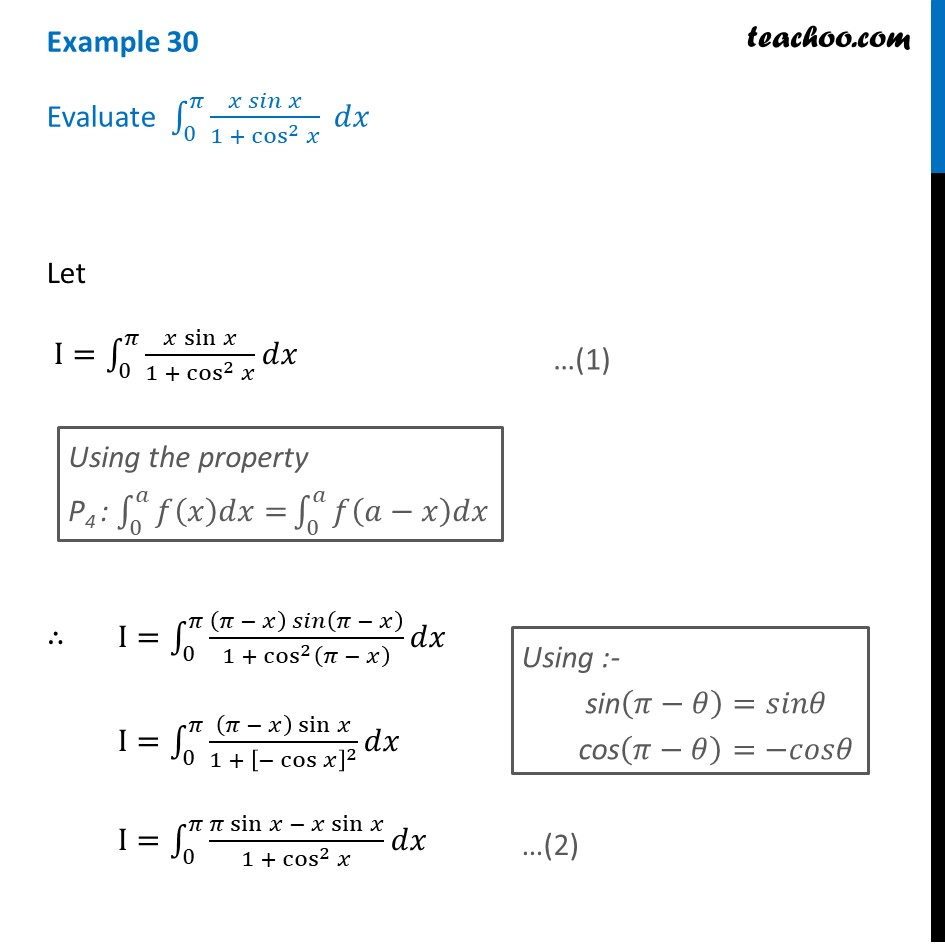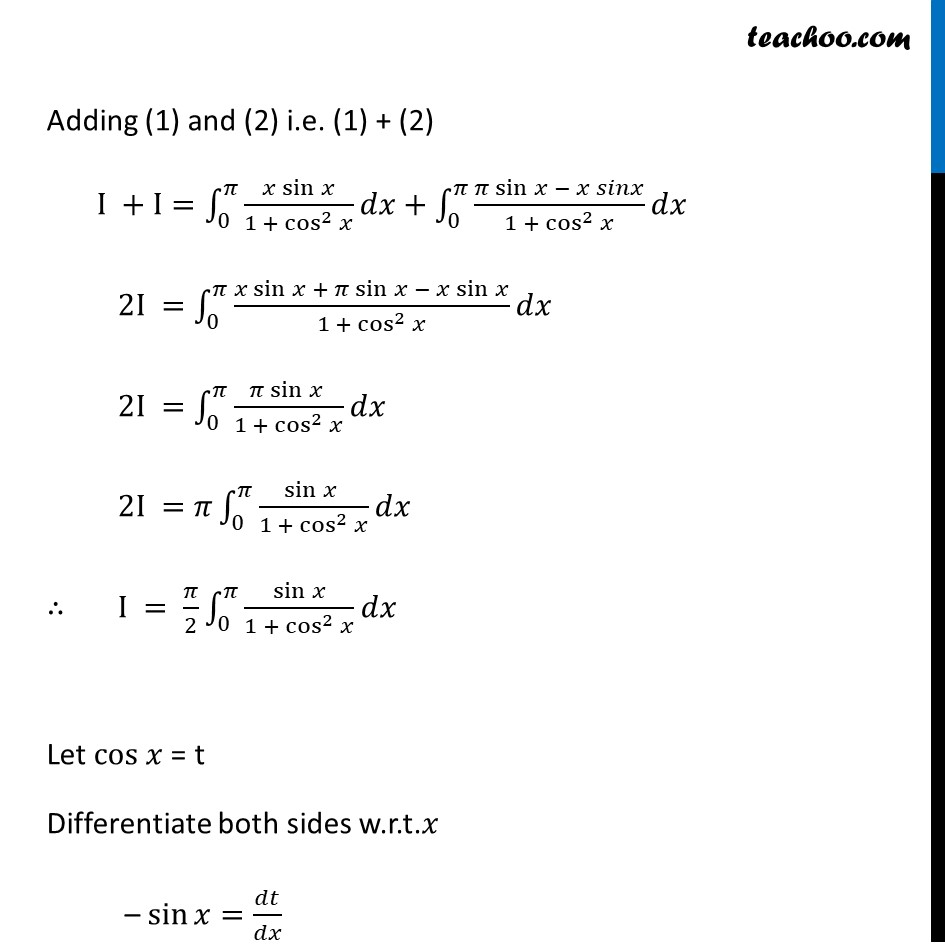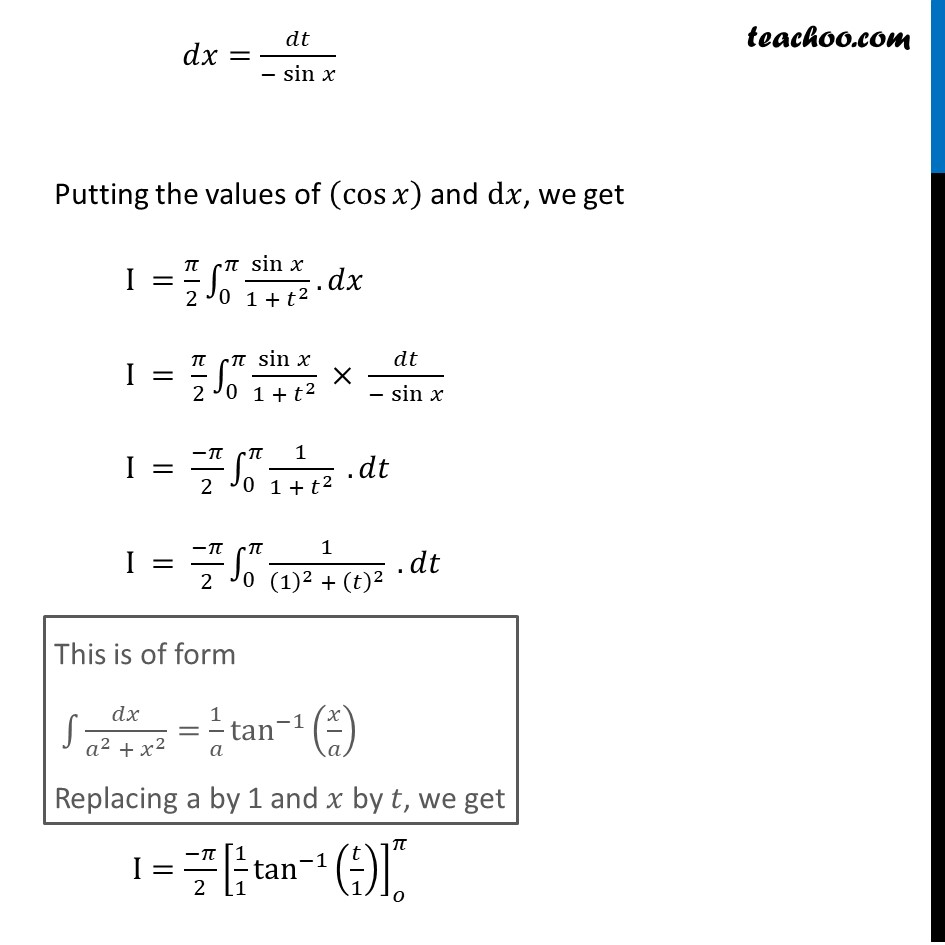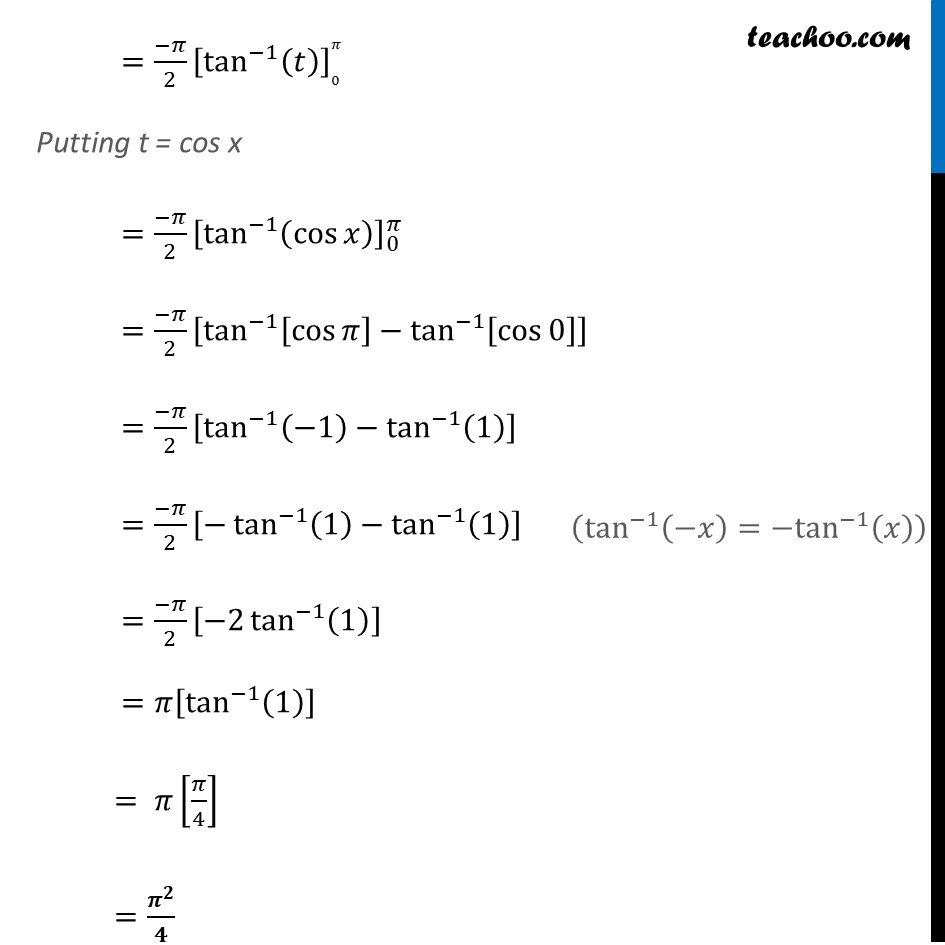Definite Integration by properties - P4

Chapter 7 Class 12 Integrals
Concept wiseLearn in your speed, with individual attention - Teachoo Maths 1-on-1 Class

### Transcript

Example 30 Evaluate ∫_0^𝜋▒(𝑥 𝑠𝑖𝑛 𝑥)/(1 + cos^2⁡𝑥 ) 𝑑𝑥 Let I=∫_0^𝜋▒(𝑥 sin⁡𝑥)/(1 + cos^2⁡𝑥 ) 𝑑𝑥 ∴ I=∫_0^𝜋▒((𝜋 − 𝑥) 𝑠𝑖𝑛(𝜋 − 𝑥))/(1 + cos^2⁡(𝜋 − 𝑥) ) 𝑑𝑥 I=∫_0^𝜋▒〖((𝜋 − 𝑥) sin⁡𝑥)/(1 + [−cos⁡𝑥 ]^2 ) 𝑑𝑥〗 I=∫_0^𝜋▒〖(𝜋 sin⁡〖𝑥 − 𝑥 sin⁡𝑥 〗)/(1 + cos^2⁡𝑥 ) 𝑑𝑥〗 Adding (1) and (2) i.e. (1) + (2) I +I=∫_0^𝜋▒(𝑥 sin⁡𝑥)/(1 + cos^2⁡𝑥 ) 𝑑𝑥+∫_0^𝜋▒(𝜋 sin⁡〖𝑥 − 𝑥 𝑠𝑖𝑛𝑥〗)/(1 + cos^2⁡𝑥 ) 𝑑𝑥 2I =∫_0^𝜋▒(𝑥 sin⁡〖𝑥 + 𝜋 sin⁡〖𝑥 − 𝑥 sin⁡𝑥 〗 〗)/(1 + cos^2⁡𝑥 ) 𝑑𝑥 2I =∫_0^𝜋▒(𝜋 sin⁡〖𝑥 〗)/(1 + cos^2⁡𝑥 ) 𝑑𝑥 2I =𝜋∫_0^𝜋▒sin⁡〖𝑥 〗/(1 + cos^2⁡𝑥 ) 𝑑𝑥 ∴ I = 𝜋/2 ∫_0^𝜋▒〖sin⁡𝑥/(1 + cos^2⁡𝑥 ) 𝑑𝑥〗 Let cos 𝑥 = t Differentiate both sides w.r.t.𝑥 – sin⁡〖𝑥=𝑑𝑡/𝑑𝑥〗 𝑑𝑥=𝑑𝑡/(−sin⁡𝑥 ) Putting the values of (cos⁡𝑥 ) and d𝑥, we get I =𝜋/2 ∫1_0^𝜋▒〖sin⁡𝑥/(1 + 𝑡^2 ).𝑑𝑥〗 I = 𝜋/2 ∫1_0^𝜋▒〖sin⁡𝑥/(1 + 𝑡^2 ) × 𝑑𝑡/(−sin⁡𝑥 )〗 I = (−𝜋)/2 ∫1_0^𝜋▒〖1/(1 + 𝑡^2 ) .𝑑𝑡〗 I = (−𝜋)/2 ∫1_0^𝜋▒〖1/((1)^2 + (𝑡)^2 ) .𝑑𝑡〗 I=(−𝜋)/2 [1/1 tan^(−1)⁡(𝑡/1) ]_𝑜^𝜋 =(−𝜋)/2 [tan^(−1)⁡(𝑡) ]_0^𝜋 Putting t = cos x =(−𝜋)/2 [tan^(−1)⁡(cos⁡𝑥 ) ]_0^𝜋 =(−𝜋)/2 [tan^(−1)⁡〖[cos⁡𝜋 ]−tan^(−1)⁡[cos⁡0 ] 〗 ] =(−𝜋)/2 [tan^(−1)⁡〖(−1)−tan^(−1)⁡(1) 〗 ] =(−𝜋)/2 [−tan^(−1)⁡〖(1)−tan^(−1)⁡(1) 〗 ] =(−𝜋)/2 [−2 tan^(−1)⁡(1) ] =𝜋[tan^(−1)⁡(1) ] = 𝜋[𝜋/4] =𝝅^𝟐/𝟒